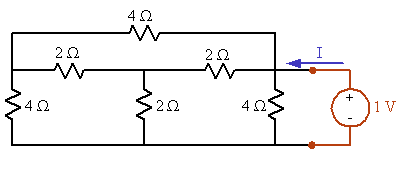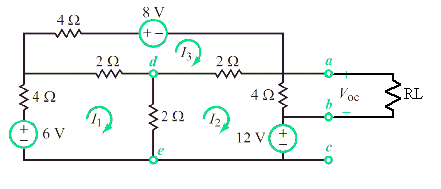# Thévenin equivalent circuit

• Engineering
Gold Member

## Homework Statement

Find the Thevenin equivalent circuit at terminals (a,b) for the circuit below.

## The Attempt at a Solution

I am having a conceptual issue for this circuit. I can't combine any of the resistors, and my gut is telling me that something about the fact that its between terminals (a,b) means that one of these resistors can be neglected and essentially erased. However, I don't understand how that works as far as what signs I should look for and what should be neglected.

I removed all the sources in an attempt to find the thévenin equivalent resistance.

#### Attachments

•3.5.png
21.1 KB · Views: 467
• 3.5 attempt 1.pdf
147.5 KB · Views: 161

phinds
Gold Member
Nothing can be neglected (your gut is wrong) and since you (as you correctly stated) cannot combine, as serial or parallel, any of the resistors, how might you go about finding the equivalent circuit ?

Gold Member
If I can't combine them, then I don't know how to find an equivalent resistance.

phinds
Gold Member
Do you know how to do loop analysis ? Do you know Kirchhoff's laws?

Gold Member
Yes I do know them. I don't see how I can use that to find the equivalent resistance when I set all the sources to zero

phinds
Gold Member
Ah, well how about delta-Y transforms, do you know them?

An alternative is to create a Thevenin equivalent for a subset of the circuit and go from there. Do it more than once if that's what it takes.

Gold Member
I was told that the 2 ohm resistor that is vertical (between d and e) has no current going through it, thus can be essentially ''erased'' from the circuit. It gives the correct answer, but I can't figure out why it is the case

phinds
Gold Member
I was told that the 2 ohm resistor that is vertical (between d and e) has no current going through it, thus can be essentially ''erased'' from the circuit. It gives the correct answer, but I can't figure out why it is the case

That certainly isn't obvious from looking at the circuit but it might be true ... only an analysis will tell, unless I'm missing something.

gneill
Mentor
Yes I do know them. I don't see how I can use that to find the equivalent resistance when I set all the sources to zero

If you suppress all the sources in the given circuit then you're left with just a network of resistors. If you then apply a known voltage across the output terminals (a - b) and determine the current that source drives into the port, you can determine the net resistance.If you intend to use loop analysis on the resulting circuit, I might recommend removing the 4 Ω resistor across the applied source first. It's a known resistance in parallel with the rest and can be "added" back later. The benefit of removing it is that it reduces the number of loops by one.

Another method for finding the Thevenin equivalent of a circuit is to tack a load resistor RL onto the output and solve for the voltage Vout across RL (leaving RL as a variable, of course).Use any method you like (loop, mesh, nodal). Take the expression you get for Vout and hammer it into the form of a voltage divider expression, since for a voltage divider formed from a Thevenin equivalent circuit with a load attached,
$$V_{out} = V_{th} \frac{RL}{RL +R_{th}}$$
Then simply pick out the terms for Vth and Rth from the result. Easy.

#### Attachments

•1 person
Gold Member
If I don't have a value for RL, how can I find Rth?

Last edited:
gneill
Mentor
Components only need share exactly two nodes in order to be in parallel. It makes no difference if other components also connect to those nodes. In the proposed circuit RL and the 4 Ω resistor in question are certainly in parallel.

gneill
Mentor
If I don't have a value for RL, how can I find Rth?

Simple. Look at the resulting expression for the voltage across RL. This is a derivation leaving RL as a symbol. The important bits (Rth and Vth) are in the rest of the expression.

Gold Member
What I did was solve for the currents. Using your labeling, I get

I1 = -1/10 A
I2 = -19/10 A
I3 = -3/2 A

Then I multiplied the 4 ohm resistor by I2 and got the Vab = -7.6 V, which is the correct answer for Vth. Now I am struggling to solve for Rth. I thought I could add a short circuit to the terminal (a,b), solve for the short circuit current, then divide Vth by Isc to get Rth

gneill
Mentor
What I did was solve for the currents. Using your labeling, I get

I1 = -1/10 A
I2 = -19/10 A
I3 = -3/2 A

Then I multiplied the 4 ohm resistor by I2 and got the Vab = -7.6 V, which is the correct answer for Vth. Now I am struggling to solve for Rth. I thought I could add a short circuit to the terminal (a,b), solve for the short circuit current, then divide Vth by Isc to get Rth

Yes, dividing Vth by the short circuit current will also give you Rth.

•1 person
Gold Member
Okay, well I redid the circuit with a short circuit, and here are my new equations

-2 -2 8 0 = -8
8 -2 -2 0 = 6
-2 8 -2 0 = -12
0 -4 0 4 = 0

then I get i4 (Isc) is -1.9 A, and divide 7.6/1.9 and it gives me 4 ohms, not the correct answer

The Electrician
Gold Member
Okay, well I redid the circuit with a short circuit, and here are my new equations

-2 -2 8 0 = -8
8 -2 -2 0 = 6
-2 8 -2 -4 = -12
0 -4 0 4 = 0

then I get i4 (Isc) is -1.9 A, and divide 7.6/1.9 and it gives me 4 ohms, not the correct answer

You forgot the item shown in red above.

•1 person
Gold Member
Okay, I see.

I am so confused because there are too many ways to find the thévenin equivalent circuits. I kind of want to master one method at a time instead of flip flop around with different methods for every problem. Are all methods applicable for all circuits, or is it just convenient to use some methods over others in particular cases?

The Electrician
Gold Member
You usually can use any network analysis method. One method may be easier for a given circuit than another.

Finding Thevenin equivalents consist of finding Vth, which is just finding the voltage across a couple of nodes, and finding Rth. Finding Rth can be done by finding the current in a short circuit across those same two nodes and dividing Vth/Isc. Or you can apply an external test current or voltage with the internal sources set to zero (except the dependent sources).

For instance, in post #9, gneill's first image shows the method of applying a test voltage. If you make the test voltage 1 volt, then your equations would become:

-2 -2 8 0 = 0
8 -2 -2 0 = 0
-2 8 -2 -4 = 0
0 -4 0 4 = 1

If you solve that system, the current in your added loop is the reciprocal of Rth; couldn't be easier.

gneill
Mentor
Okay, I see.

I am so confused because there are too many ways to find the thévenin equivalent circuits. I kind of want to master one method at a time instead of flip flop around with different methods for every problem. Are all methods applicable for all circuits, or is it just convenient to use some methods over others in particular cases?

In general the idea is to find the open source voltage and output resistance of the network in question. How one goes about doing that for any particular circuit is a matter of applying favorite techniques. If you're really comfortable with loop analysis, use it. Same goes or nodal analysis. All the tools one has learned to use (parallel/series simplification, Ohm's Law, KVL, KCL, loop, nodal, mesh,...) can come in handy. Choosing the best technique for a given circuit is largely a matter of experience gained through practice.

For circuits comprised of resistors and fixed sources only, the open circuit voltage and network reduction method always works. Finding the short circuit current can replace the network reduction (essentially finding the Norton equivalent and using the Norton <---> Thevenin equivalency).

When controlled sources are present it can be trickier. One cannot simply suppress them as you do fixed sources to find the circuit equivalent resistance; There must be some power source active in the circuit in order to "stimulate" the controlled source behavior. Here the short circuit current method is useful if the controlled sources in the circuit are activated by fixed supplies internal to the circuit. If not you need to apply an external source to the output to get things working.

My own favorite method involves placing a load on the circuit and solving (symbolically) for the voltage across the load. Since a Thevenin source with a load is just a voltage divider scenario, one can pick out the Thevenin voltage and resistance directly from the resulting expression for Vout, thus finding both values at the same time.